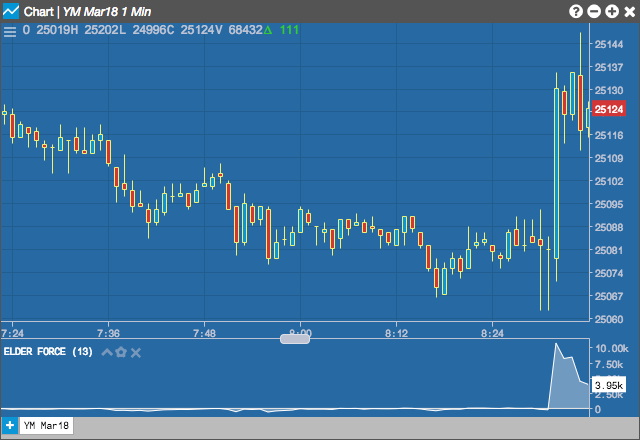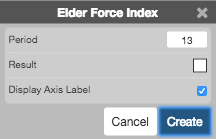Charts

# Elder Force Index (EFI)

The Elder Force Index indicator is used to measure the power driving a movement in the price. It tries to highlight potential price reversals and corrections by analyzing the direction, length, and volume driving the price movement.## Configuration Options• Period: Number of bars to use in the calculations.
• Color Selectors: Colors to use for graph elements.
• Display Axis Label: Whether to display the most recent value on the Y axis.

## Formula

$EFI = (Close_{current\;period} - Close_{previous\;period})\times Volume_{current\;period}$

$EFI_{n-period} = Moving\;Average\;of\;n-periods\;of\;EFI$